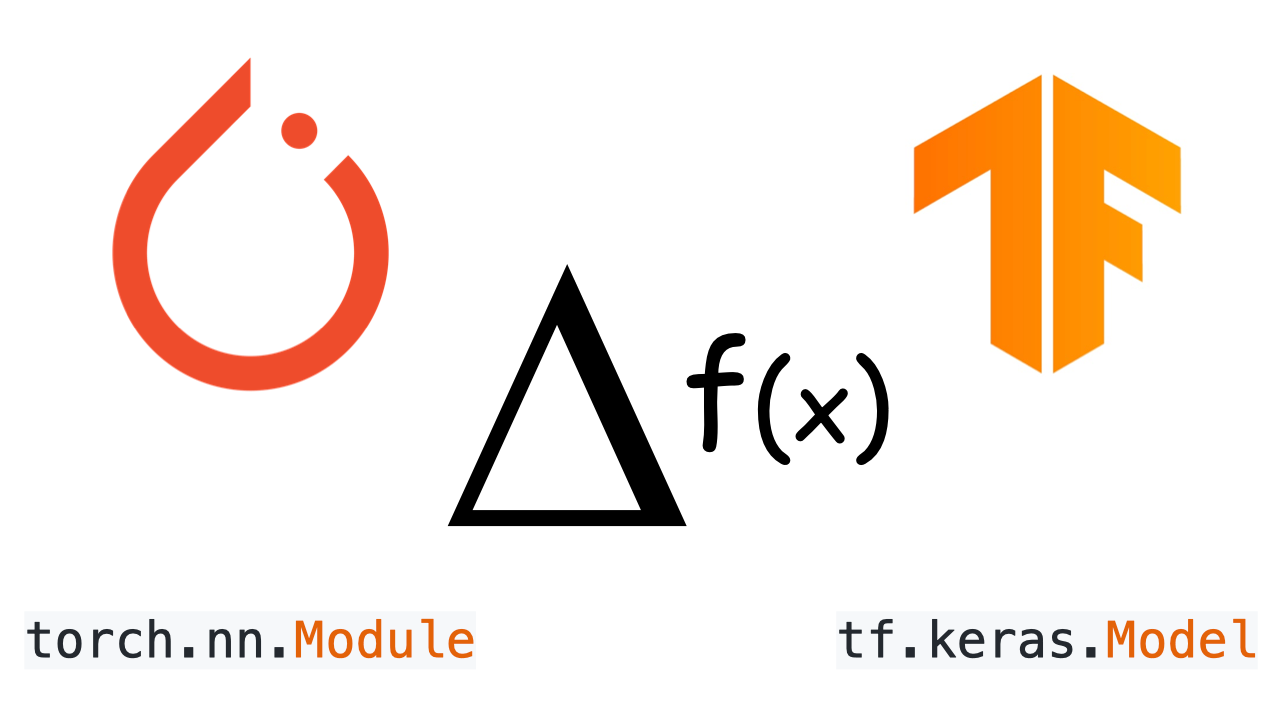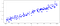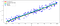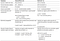# A tale of two frameworks: PyTorch vs. TensorFlow

## Comparing auto-diff and dynamic model sub-classing approaches with PyTorch 1.x and TensorFlow 2.xSource: Author

The data science community is a vibrant and collaborative space. We learn from each other’s publications, debate ideas on forums and online outlets, and share lots (and lots) of code. A natural side effect of this collaborative spirit is the high likelihood of encountering unfamiliar tools used by our colleagues. Because we don’t work in a vacuum, it often makes sense to gain familiarity with several languages and libraries in a given subject area in order to collaborate and learn most effectively.

It’s no surprise, then, that many data scientists and Machine Learning engineers have two popular Machine Learning frameworks in their toolboxes: TensorFlow and PyTorch. These frameworks — both in Python — share many similarities, but also diverge in meaningful ways. These differences, such as how they handle APIs, load data, and support specialized domains, can make alternating between the two frameworks cumbersome and inefficient. And that’s a problem given how common both of these tools are.

Therefore, this article aims to illustrate the differences between PyTorch and TensorFlow by focusing on the basics of creating and training two simple models. In particular, we’ll cover how to use dynamic subclassed models with the Module API from PyTorch 1.x and the Module API from TensorFlow 2.x. We’ll look at how auto-diff can be used by these frameworks, too, to provide very naïve implementations of gradient descent.

## But first, data

Because we are focusing on the core of auto-diff/auto-grad functionality (which, as a refresher, is the capacity to automatically extract the derivative of a function and apply the gradients with respect of some parameters in order to optimize these parameters using gradient descent) we can start with the simplest possible model, a linear regression. We can use the numpy library to generate some linear data with a bit of random noise, and then run our models on that dummy data set.Source: Author

## The models

Once we have data, we can implement a regression model from scratch in both TensorFlow and PyTorch. For simplicity, we won’t initially use any layers or activators, just define two tensors, w and b, that represent the weights and bias of the linear model y = wx + b.

As you can see below, other than a few differences in the API names, the class definitions for the two models are nearly identical. The most significant difference is that PyTorch requires an explicit Parameter object to define the weights and bias tensors to be captured by the graph, whereas TensorFlow is able to automagically capture those same parameters. Indeed, PyTorch parameters are tensor subclasses that have a special property when used with the Module API: They automatically add self to the list of Module parameters, so self appears, for example, in the parameters() iterator.

Both frameworks extract everything needed to generate the graph from this class definition and its execution methods (__call__ or forward) and, as we see below, calculate the gradients needed to implement backpropagation.

## Building the training loop, backpropagation, and optimizers

With these simple TensorFlow and PyTorch models established, the next step is to implement the loss function, which in this case is just means squared error. We can then instantiate the model classes and run the training loop for a few cycles.

Again, because we’re focusing on the core auto-diff/auto-grad functionality, the aim here is to build a custom training loop using TensorFlow and PyTorch–specific auto-diff implementations. These implementations calculate gradients for the simple linear function and manually optimize the weight and bias parameters with a naïve gradient descent optimizer, essentially minimizing the loss computed between the real point and the prediction using the differentiable function at each point.

For the TensorFlow training loop, I explicitly used the GradientTape API to track the forward execution of the model and step-wise loss calculations. I used the gradients from GradientTape to optimize the weights and bias parameters. PyTorch provides a more “magical” auto-grad approach, implicitly capturing any operations on the parameter tensors and providing the gradients to use for optimizing the weights and bias parameters without having to call another API. Once I have the weights and bias gradients, implementing the custom gradient descent method on both PyTorch and TensorFlow is as simple as subtracting the weight and bias parameters from these gradients, multiplied by a constant learning rate.

Note that because PyTorch implements auto-diff/auto-grad automatically, it’s necessary to explicitly call the no_grad API right after we computed the backward propagation. This instructs PyTorch not to compute the gradients for the update operation of the weights and bias parameters. We also need to explicitly zero-out the previously automatically-computed gradients, computed in the forward operation, to stop PyTorch from automatically accumulating gradients in all batch and cycles iterations.

## PyTorch and TensorFlow models reusing available Layers

Now that I’ve shown how to implement linear regression models from scratch in PyTorch and TensorFlow, we can look at how to reimplement the same models using Dense and Linear layers, respectively, from the TensorFlow and PyTorch libraries.

## TensorFlow and PyTorch dynamic models with existing layers

You’ll notice in both model initialization methods that we are replacing the explicit declaration of the w and b parameters with a Dense layer in TensorFlow and a Linear layer in PyTorch. Both of these layers implement the linear regression, and we’ll instruct them to use single weight and bias parameters in place of the explicit w and b parameters used before. Dense and Linear implementations will internally use the same tensor declarations we used before (respectively tf.Variable and nn.Parameter) to allocate these tensors and associate them with the model parameters list.

We’ll also update the call / forward methods of these new model classes to replace the manual linear regression computation with the execution of the Dense / Linear layers.

## Training with available optimizers and loss functions

Now that we have re-implemented our TensorFlow and PyTorch models using existing Layers, we can focus on how to build more optimized training loops. Rather than using our previous naïve implementation, we’ll use the native Optimizers and Loss Functions available from these libraries.

We’ll continue to use the auto-diff/auto-grad functionalities observed before, but this time with a Standard Gradient Descent (SGD) optimization implementation alongside a standard loss function.

## TensorFlow training loop with easy Fit method

In TensorFlow, fit() is a very powerful and high-level method for training a model. It allows us to replace a manual training loop with a single method that specifies the hyper-tuning parameters. Before calling fit() we’ll compile our model class using the compile() method, and then pass a gradient descendent optimizer and a loss function to use for the training.

You’ll notice that in this case we will reuse the methods from the TensorFlow library as much as possible. In particular, we will pass a standard Stochastic Gradient Descent (SGD) optimizer and a standard Mean Absolute Error loss function implementation (mean_absolute_error) to the compile method. Once the model is done compiling, we can finally call the fit method to fully train our model. We’ll pass the data (x and y), the number of epochs, and the batch size to use on each epoch.

## TensorFlow training loop with custom loop and SGD Optimizer

In the following code snippet, we will implement another custom training loop for our model, this time reusing the loss functions and optimizers provided by the TensorFlow library as much as possible. You’ll notice that our former custom Python loss function is replaced with the tf.losses.mse() method. Instead of manually updating the model parameters with the gradients, we initialize a tf.keras.optimizers.SGD() optimizer. Calling the optimizer.apply_gradient() and passing a list of weight and bias tuples will update the model parameters with gradients.

## PyTorch training loop with custom loop and SGD Optimizer

As in the previous TensorFlow code snippet above, the following code snippet implements a PyTorch training loop for our new model by reusing loss functions and optimizers provided by the PyTorch library. You’ll notice we’ll replace our former custom Python loss function with the nn.MSELoss() method and initialize a standard optim.SGD() optimizer with the list of our model’s learning parameters. As previously illustrated, we will instruct PyTorch to obtain the associated gradients for each parameter tensor from the loss backward propagation (loss.backward()), and finally, we can easily update the new standard optimizer with all parameters associated with the gradients by calling the optimizer.step() method. The way that PyTorch makes automatic associations between tensors and their gradient allows the optimizer to retrieve the tensors and gradients to update them with the configured learning rate.

## Results

As we saw, the TensorFlow and PyTorch auto-diff and Dynamic sub-classing APIs are very similar, even in the way they use standard SGD and MSE implementations. Naturally, both models also gave us very similar results.

In the code snippet below, we use Tensorflow’s training_variables and PyTorch’s parameters methods to get access to the models’ parameters and plot the graph of our learned linear functions.Source: Author

## Conclusion

Both PyTorch and the new TensorFlow 2.x support Dynamic Graphs and auto-diff core functionalities to extract gradients for all parameters used in a graph. You can easily implement a training loop in Python with any loss functions and gradient descendent optimizers. In order to focus on real core differences between the two frameworks, we simplified the example above by implementing our own simple MSE and naïve SGD.

However, I strongly suggest that you reuse optimized and specialized code available on these libraries before implementing any naïve code.

The table below summarizes all the differences we noted in the sample code above. I hope it can serve as a useful reference for when you need to switch between these two frameworks.Source: Author

## Data Science at Microsoft

Lessons learned in the practice of data science at…

## Data Science at Microsoft

Lessons learned in the practice of data science at Microsoft.

Written by

## Jacopo Mangiavacchi

Microsoft Senior Data Scientist — Google Machine Learning Developer Expert (ML GDE) — Former  + IBM Senior Architect and Engineer## Data Science at Microsoft

Lessons learned in the practice of data science at Microsoft.

## More From Medium

Medium is an open platform where 170 million readers come to find insightful and dynamic thinking. Here, expert and undiscovered voices alike dive into the heart of any topic and bring new ideas to the surface. Learn more

Follow the writers, publications, and topics that matter to you, and you’ll see them on your homepage and in your inbox. Explore

If you have a story to tell, knowledge to share, or a perspective to offer — welcome home. It’s easy and free to post your thinking on any topic. Write on Medium